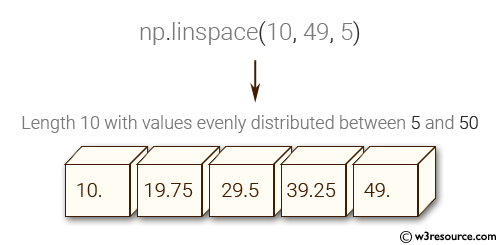﻿ NumPy: Create a vector of length 10 with values ​​evenly distributed between 5 and 50 - w3resourceNumPy: Create a vector of length 10 with values ​​evenly distributed between 5 and 50

NumPy: Basic Exercise-21 with Solution

Write a NumPy program to create a vector of length 10 with values ​​evenly distributed between 5 and 50.

Sample Solution :

Python Code :

import numpy as np
v = np.linspace(10, 49, 5)
print("Length 10 with values evenly distributed between 5 and 50:")
print(v)

Sample Output:

Length 10 with values evenly distributed between 5 and 50:
[ 10.    19.75  29.5   39.25  49.  ]

Pictorial Presentation:Python Code Editor:

Have another way to solve this solution? Contribute your code (and comments) through Disqus.

What is the difficulty level of this exercise?

﻿